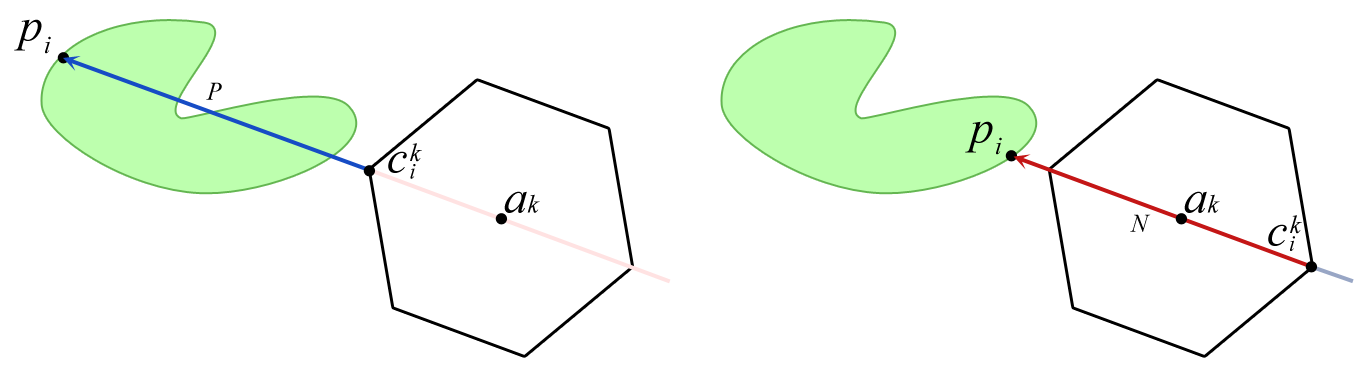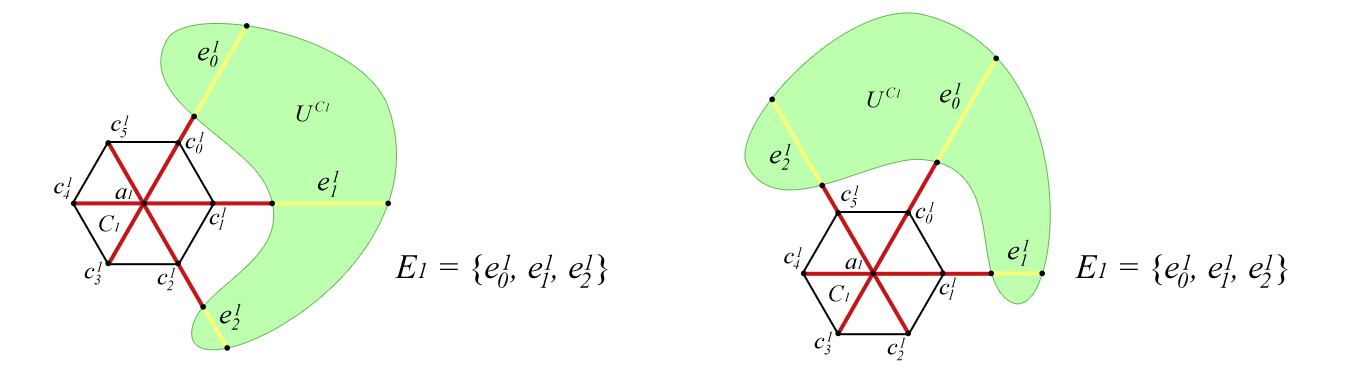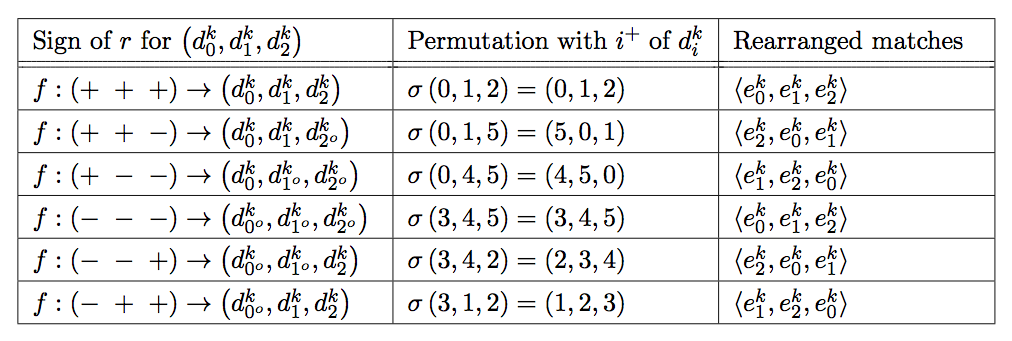## Saturday, February 6, 2016

### A scalable geometrical model for muscle and tendon units: An algorithmic solution on how to fit a template muscle on a high resolution muscle mesh

The reason for this blog post is to share a bit on a particular hard problem that I've encountered during my Master's thesis with a broader audience. I will try my best to write it in plain English, but as the problem is complex expect this to be a lengthy post with domain specific terminology.

## The problem

Mammalian muscle comes in all shapes and sizes, the material properties are also heterogenous and the space in which muscles are usually situated are tight and intertwined with other components of the organism. On the other hand you have models in biomechanics that try to simulate the physical and mechanical work of muscles. These are usually represented as single piecewise line segments for several reasons. Most importantly it works and it's simple. In addition, the accuracy of these models is under constant research and is constantly improving. For the thesis we wanted to explore the possibility of combining the best of both worlds, i.e. having a trial-and-tested biomechanics model surrounded by a geometrical shape that represents a muscle in three dimensions. Applicable for uses in computer animation where realism plays a bigger role. However, the problem required for the solution to perform in real-time, with a refresh rate of at least 60Hz. This meant having a bottom-up approach in terms of resolution, starting with a simple segmented-cylinder as the base template and scaling the resolution upwards by adding more points (points are also referred here as vertices). This last criterion is actually part of the proposed solution as it brings with it the possibility to adapt the level-of-detail based on the application where its being used. For instance, in your real-time engine if you have a lot of objects and interactions running at the same time you might want to consider reducing the resolution. Conversely, if the system or scene permits it you can opt to increase the resolution in order to have a more accurate representation while still maintaining a proper frame rate. You have to also think that the muscle shapes are constantly deforming during runtime, using the biomechanics model as their centre and base. The piecewise line in the biomechanics model is referred to as the muscle's line of action and resides inside the geometrical shape. This association between the model and geometry is basically the process commonly known as 'skinning' in the computer animation universe. One additional note for this specific setup: the final position of the vertices are calculated using forward kinematics, starting at the "top" side going through to the "bottom" of the muscle, also known as the origin and insertion points of the action line.

## The solution (TL;DR)

In case of TL;DR, the solution to the problem is also presented in this five minute video:

And/or you can keep on reading for the long version.

## The solution (long version)

This is the lengthy part of the post as it delves into the more algorithmic aspects of the solution on how to fit a template muscle shape onto a high resolution muscle shape in order to achieve more realism. As mentioned in the previous paragraph, the basic idea starts by adding some volume through geometry. This is done with a template shape in the form of a closed segmented cylinder with bisecting cross sections. The cylinder also includes an extra cross section to separate the muscle from the tendon compartment. Although this by itself comes with its own set of issues, the implementation is nevertheless there to be improved upon :)

Now unto the nitty gritty. At the beginning, when the character is configured in the popular t-pose, we transform a high resolution muscle shape (geometrical shapes are also referred here as a meshes) as much as possible to coincide with the routing of its associated action line, in position, orientation, and scale. Next, at each centre vertex of the action line we sample points in the same plane as its bisecting cross section to find out where in space the template cylinder is configured relative to the target mesh. This is done using a technique known as ray casting or ray sampling, basically "shooting" rays until it intersects a triangle of the high resolution muscle mesh. The decision to opt for this approach is an important one, and the reason as to why requires some explanation. Popular 3D modelling software often have tools to split and manipulate meshes, one such technique is known as "knifing" where you cut the mesh on a plane that you define with an input device such as your mouse. You might ask yourself, why can't I simply cut the target mesh, well the reasons are twisting and control.

### Let's not twist again

You see, splitting a mesh adds extra vertices on the edges of the triangle that is being cut. The position of these extra points will not match the expected position of the points that define the surface of the segmented cylinder, even in case you have the same number of vertices in both cross sections. Let's take a cross section of a particular segmented cylinder, say my template muscle has cross sections with six vertices, forming a hexagon. When I cut the target mesh in the same plane as the hexagon, I can get more, less, or an equal number of vertices on the target mesh, depending on the target muscle geometry and the position of the plane where the cut is taking place. Say I get more vertices than the template cross section, I can still collapse edges to reduce the number of vertices until I get to a total of six. Still, these new points will not be able to correspond to the same vertices of the template mesh and if you repeat this process for every cross section you would eventually get a twisted (or zigzag) segmented cylinder.

### Control Freak

Because we wanted to have a systematic way to the control the number of vertices, we developed the technique to be able to add or reduce the same number of vertices for every cross section and for every action line. The technique can do this in both directions by (1) adding more cross sections and (2) adding more points on every cross section. So one solution that stuck is the sampling of target meshes with rays. With this solution we can keep the control as we can define exactly how many vertices there would be, we can get a correspondence with a target mesh because of the sampling method explained in the next paragraph thereby eliminating twisting, and most importantly it allows us to determine where the template shape resides in space with respect to its corresponding target mesh. From here we can further determine if we have to move the action line of the template shape inside the target mesh, or just let the template shape approximate the shape of the target mesh in equal angles. (Note: if you have any other ideas I would like to hear them.)

## Mesh Sampling

We start by casting rays, starting from all surface points of each template cross section and on the same plane as each cross section. In addition, there are two rays being cast for every point. These are cast in the directions of a surface point minus a centre point and its negative counterpart, this gives us rays that are cast outside of the template shape as well as rays that are cast inside the shape, shown in Figure 1.Figure 1: Blue arrows are rays cast in the positive direction, red arrows are rays cast in the negative direction.

Once we have collected all intersection points, we have to disregard a couple of points by introducing two "preference" rules. These are meant to cope with the fact that each triangle in computer graphics has two faces, namely a triangle can be either front or back-faced and so this extra piece of information can be used to determine if the ray is hitting the triangle from the outside or from the inside of the target shape. The first rule, shown in Figure 1, is to prefer rays being cast in the outside (positive) direction instead of the negative (inside) direction. The second rule is that for every outside intersection test, the furthest found intersection point of a back-faced triangle is preferred, while for every inside intersection test the closest found intersection point of a front-faced triangle is preferred. These two rules of preference allow us to handle the three possible configuration states, namely:

1. The high resolution mesh lies completely outside of the template cross section.
2. The high resolution mesh lies completely inside of the template cross section.
3. The high resolution mesh lies partly outside of the template cross section.

Figure 2 provides two visual examples that highlights the use of the second rule.Figure 2: Outside rays take the furthest intersection from a back-face triangle, while inside rays take the closest intersection from a front-face triangle.

Once the intersection points are established we have to test to see in which configuration state a particular cross section resides, partly inside, completely inside or completely outside of the high resolution mesh. This determination is carried out by a process we call "match detection".

## Permuting Matches

Once we have a set of matches we have to permute (or rearrange) the order. This is necessary in order to form a simple polygon of the set of matches so we can determine a new "centre" for every centre point of the action line of the cylindrical muscle shape. It just so happens that if you use every time a normal order, say you start from index zero, you will get a different shape due to different relative configurations as shown in Figure 3.Figure 3: A use case showing the two configuration states where one starts at index 5 the other at index 0, the drawing should start from index 5 in the right case and not at index 0.

What we would like to achieve is a way to permute the indices so that it always starts with a match at the "start" of the shape, consequently "winding" through the set of matches, and reaching the "end" of the shape. One example of an end result after running the permutation algorithm for a particular set of six vertices is given in Table 1.Table 1: The first column shows the mapping of the radius sign with a particular surface point that was translated after intersection tests. The second column shows the result of the permutation algorithm, and the third column shows the newly formed sequence that contain the same pattern for the first half and second opposing half.

Note that because we are dealing with pairs of indices we only have to permute half and we can always refer to the opposing index by adding it with half of the size of the index store. So in case of a hexagon, the opposing index 0 is 3. A snippet of the permutation algorithm in C++ code is as follows:

``````// Sort first element of std::pair<int, int>
struct sort_pred {
bool operator()(const std::pair<int,int> &left, const std::pair<int,int> &right) {
return left.first < right.first;
}
};

void Enhansor::translateCrossSection(int seg_index)
{
// Keep count for each matched j-index, do intersection tests at the end
std::vector<std::pair<int, int> > matches;

// Localize parameter
int i = seg_index;

// Temp int for getting the half of the cross-section shape
int half_cs = this->target_lat / 2;

// Just process half of the shape, e.g. hexagon = 3
// The standard j<x is used instead of j<=x for consistency in for-loops.
for(int j = 0; j < half_cs; j++)
{
// Check if there is a displacement
if(this->intersection[i][j].intersect_p != Vector3::ZERO)
{
// It was a positive intersection (same side)
if(this->intersection[i][j].same_side == 1)
{
matches.push_back(std::pair<int, int>(j, j + half_cs));
}
else // It was a negative intersection (opposite side = positive)
{
matches.push_back(std::pair<int, int>(j+half_cs, j));
}
}
}

// For remembering the matched pairs of intersected points
int k = (int)matches.size();

// Sort the vector based on the following rule:
// When a difference is encountered that is larger than 1
// the index m+1 gets shifted to the front of the set
// we count the amount of shifts as well (n_shifts)
// if another point is encountered after this match that is
// larger than 1 we then shift this index to n_shifts in the set
// Examples:
// we have 3,4,2 becomes 2,3,4 (for hexagons)
// we have 0,1,6,7 becomes 6,7,0,1 (for octagons)
// we have 0,1,7,8,9 becomes 7,8,9,0,1 (for decagons)
// This rule makes sure that the order is following the
// intersection-matches continuously in the high resolution mesh

// Sort matches ascending on first element.
std::sort(matches.begin(), matches.end(), sort_pred());

int temp_mindex = matches.first;
int n_shifts = 0;
std::pair<int, int> temp_pair;
std::vector<std::pair<int, int> > temp_matches;
temp_matches = matches;

for(int m = 1; m < k; m++)
{
if((matches[m].first - temp_mindex) != 1)
{
// Remove element and add it to the front
temp_pair = std::make_pair(matches[m].first, matches[m].second);
temp_matches.erase(temp_matches.begin() + m);
temp_matches.insert(temp_matches.begin() + n_shifts, temp_pair);
// Keep count of number of shifts
n_shifts++;
}
temp_mindex = temp_matches[m].first;
}

// Copy back into the original set
matches = temp_matches;

//... code with lots of intersection tests not shown here... //

// Clear the contents to let runtime clear some memory
matches.clear();
}
``````

Once the permutation is complete, a centre point can easily be determined by either taking the average of four surface points from the middle two matches in case the set of matches has an even size or taking half of the middle match in case the set of matches has an odd size, as shown in Figure 4.

The technique is also able to increase the resolution in a separate thread just to find a new centre point that is inside, while keeping the original requested resolution as is (a necessity for tricky boomerang-like muscle shapes). One nice thing to mention about the problem domain is that fortunately for typical animations such as walking, running, crawling, etc., donut-shaped muscles are not required to be rendered (whew).

I hope that this blog post gave a compact but clear idea of the most important aspect of the solution that tackles the problem of fitting a simple cylinder shaped muscle with an underlying biomechanics model unto a high resolution shaped muscle. It's a very specific problem with a very specific set of requirements, and sharing my solution through a blog post would perhaps allow others to expand and improve on the idea or provide alternative ways to tackle the same or similar issues. That's what I love about science! The work was also published and can be found in the paper: A scalable geometrical model for musculotendon units, which had a runtime of 135Hz. Feel free to drop a comment below.